Next: 4. Simulation Application Up: 3.7 Generation and Recombination Previous: 3.7.4 Band-to-Band Tunneling

Subsections

## 3.7.5 Impact Ionization

The impact ionization (II) models support both the drift-diffusion (DD) and the hydrodynamic (HD) transport models, therefore, electric field dependent DD II models and carrier temperature dependent HD II models are used in MINIMOS-NT.

### 3.7.5.1 Drift-Diffusion Impact Ionization

In DD simulation the model from  is used to calculate the II generation rates for electrons and holes, respectively. The overall generation rate is the sum of these two generation rates and can be expressed as a negative recombination rate.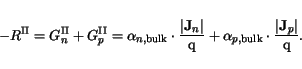(3.152)

The ionization coefficients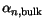and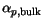are expressed by Chynoweth's law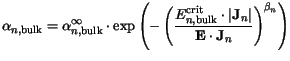(3.153)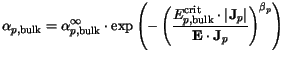(3.154)

The default values are summarized in Table 3.38.

Table 3.38: Parameter values for DD impact ionization model
 Material[m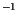]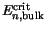[V/m][m]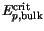[V/m]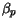Reference Si 7.03e7 1.231e8 1.0 1.528e8 2.036e8 1.0 Ge 1.55e9 1.560e8 1.0 1e9 1.28e8 1.0  GaAs 3.5e7 6.85e7 2.0 3.5e7 6.85e7 2.0  GaP 4.0e7 1.18e8 2.0 4.0e7 1.18e8 2.0 

To account for surface effects, the surface ionization rates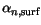and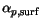can deviate from the bulk rates. The electron surface ionization rate is calculated in a similar way (analog for holes)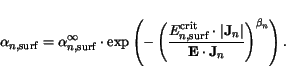(3.155)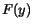is given by (3.120) and depending on the surface distance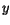describes a smooth transition between the surface and bulk generation rates. The parameter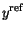denotes a critical length. The final surface dependent ionization rate reads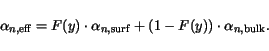(3.156)

The effect is considered only for Si and the following values are used:

Table 3.39: Parameter values for surface DD impact ionization model
 Material[m]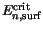[V/m][m][V/m][nm] Si 1.03e7 1.50e8 4.0e8 3.0e8 10

### 3.7.5.2 Hydrodynamic Impact Ionization

In a HD simulation, the carrier temperatures are used as parameters in the hydrodynamic impact ionization model. The implemented equation for the electron generation rate depending on the concentration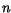and the bandgap energy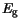[191,192] reads (analog for holes)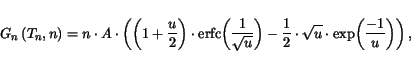(3.157)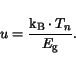(3.158)

The prefactor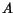depends on the carrier and lattice temperatures and the local bandgap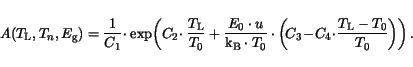(3.159)

The variables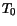and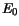correspond to 300 K and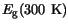, respectively.

Table 3.40: Parameter values for HD impact ionization model
 Material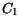[s]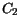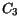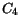Si 9.531e-9 3.823 0.346333 0.0922

The overall generation rate is the sum of the electron and hole generation rates, and is equal to a negative recombination rate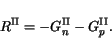(3.160)

Another simple, but very practical model is available for modeling the impact ionization rate in all semiconductors. It reads for electrons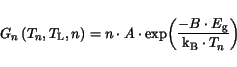(3.161)

and, respectively, for holes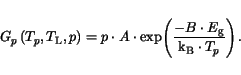(3.162)

The default values recommended for the simple HD II model are summarized in the following table:

Table 3.41: Parameter values for HD impact ionization model
 Material[s]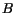Si&Ge 1e13 0.92 III-Vs 1e13 1.0

This model has been already successfully applied in simulation of GaAs-based and InP-based HEMTs [193,194]. However, it has not been applied in simulation of III-V HBTs yet.Next: 4. Simulation Application Up: 3.7 Generation and Recombination Previous: 3.7.4 Band-to-Band Tunneling
Vassil Palankovski
2001-02-28